### Ordinary Differential Equations: Classification of ODEs

### Classification of ODEs

#### Order

The order of an ODE is the order of the highest derivative appearing in the equation. For example, the following equation (Newton’s equation) is a second-order ODE: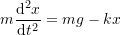while the beam equation is a fourth-order ODE: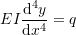#### Linear vs. Nonlinear

An ODE is linear if it can be written as the linear combination: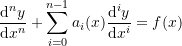where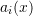and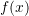are functions of the independent variable. All the examples above are considered linear ODEs.
The following are two examples of nonlinear ODEs withbeing the dependent variable andbeing the independent variable: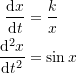#### Homogeneous vs. Nonhomogeneous

A homogeneous ODE is an equation whose every term contains either the dependent variable or one of its derivatives. For example, Newton’s second law applied to a spring without the gravitational term is a linear homogeneous ODE: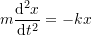If one of the non-zero terms in the ODE contains neither the dependent variable nor any of its derivatives, the equation is nonhomogeneous. As an example, if the gravitational term is added to Newton’s second law, then, the equation becomes nonhomogeneous:The free term (the term devoid of the dependent variables and any of its derivatives) is called the source term.

A linear homogeneous ODE has the following form: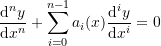while a linear nonhomogeneous ODE has the form:The termis called the source term. The solution to a linear homogeneous equation is called the complementary solution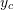while the solution when a source term appears in the equation is the sum of the complementary solutionand the particular solution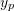(which is particular to the source term). For example, the solution to Newton’s second law without the gravitational term has the form: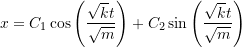while the solution when the gravitational term appears has the form: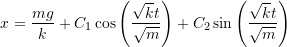The particular solution is the term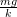.

#### IVP vs. BVP

Depending on the boundary conditions, an ODE can be classified as either an Initial Value Problem (IVP) or a Boundary Value Problem (BVP). An initial value problem is an ODE given with initial conditions of the dependent variable and its derivative at a particular value of the independent variable. This usually applies to dynamic systems whose independent variable is time. For example, Newton’s second law of motion is an initial value problem because the initial value at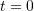of the displacement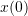and the velocity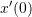are required to reach a solution. The initial values lead to a particular path that is a function of time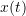.

A boundary value problem is an ODE given with boundary conditions at different points. This usually applies to static systems whose independent variable is position. For example, the Euler-Bernoulli beam deflection equation is a boundary value problem. The boundary conditions of the displacement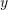, the rotation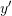, and the third and fourth derivatives, are usually given at boundary points on the beam which will then dictate the equilibrium deflection of the beam as a function of position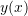.